## 如何將一個文件夾中的多個文本文件導入一個工作表？

1.啟用要導入文本文件的工作簿，然後按 Alt + F11鍵 啟用鍵 Microsoft Visual Basic for Applications 窗口。

2。 點擊 插入 > 模塊，將下面的VBA代碼複製並粘貼到 模塊 窗口。

VBA：將多個文本文件從一個文件夾導入到一張工作表

``````Sub Test()
'UpdatebyExtendoffice6/7/2016
Dim xWb As Workbook
Dim xToBook As Workbook
Dim xStrPath As String
Dim xFileDialog As FileDialog
Dim xFile As String
Dim xFiles As New Collection
Dim I As Long
Set xFileDialog = Application.FileDialog(msoFileDialogFolderPicker)
xFileDialog.AllowMultiSelect = False
xFileDialog.Title = "Select a folder [Kutools for Excel]"
If xFileDialog.Show = -1 Then
xStrPath = xFileDialog.SelectedItems(1)
End If
If xStrPath = "" Then Exit Sub
If Right(xStrPath, 1) <> "\" Then xStrPath = xStrPath & "\"
xFile = Dir(xStrPath & "*.txt")
If xFile = "" Then
MsgBox "No files found", vbInformation, "Kutools for Excel"
Exit Sub
End If
Do While xFile <> ""
xFile = Dir()
Loop
Set xToBook = ThisWorkbook
If xFiles.Count > 0 Then
For I = 1 To xFiles.Count
Set xWb = Workbooks.Open(xStrPath & xFiles.Item(I))
xWb.Worksheets(1).Copy after:=xToBook.Sheets(xToBook.Sheets.Count)
On Error Resume Next
ActiveSheet.Name = xWb.Name
On Error GoTo 0
xWb.Close False
Next
End If
End Sub``````

3。 按 F5 顯示對話框，然後選擇一個文件夾，其中包含要導入的文本文件。 看截圖：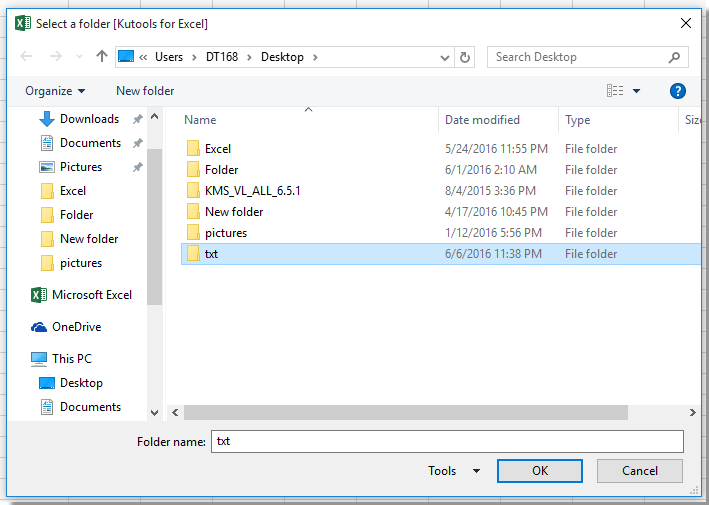4。 點擊 OK。 然後，文本文件已作為新工作表分別導入到活動工作簿中。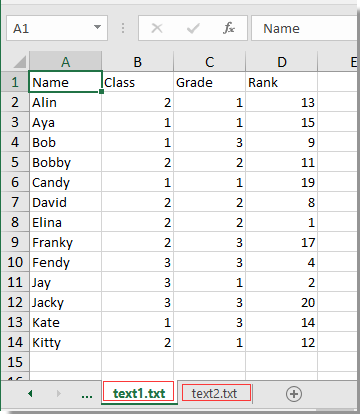與以上 300 方便的功能，使您的工作更加輕鬆。 免費下載免費全功能 30 天

Kutools for Excel，請執行以下操作：

1.選擇要導入文本文件的單元格，然後單擊 Kutools 加 > 進出口 > 在光標處插入文件。 看截圖：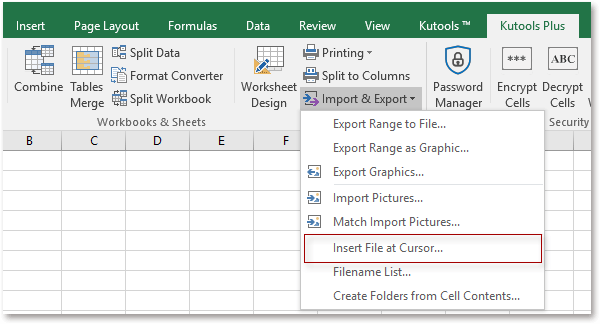2.然後彈出一個對話框，單擊 瀏覽 顯示 選擇一個文件 插入單元格光標位置對話框中，然後選擇 文本文件 從下拉列表中，然後選擇要導入的文本文件。 看截圖：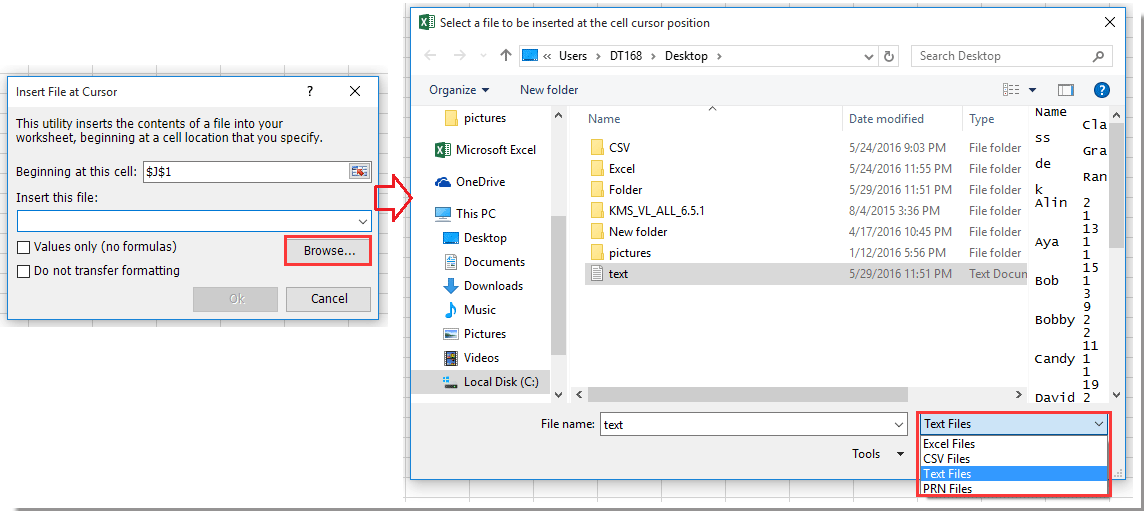3。 點擊 已提交 > Ok，並且已在光標位置插入了指定文本文件，請參見屏幕截圖：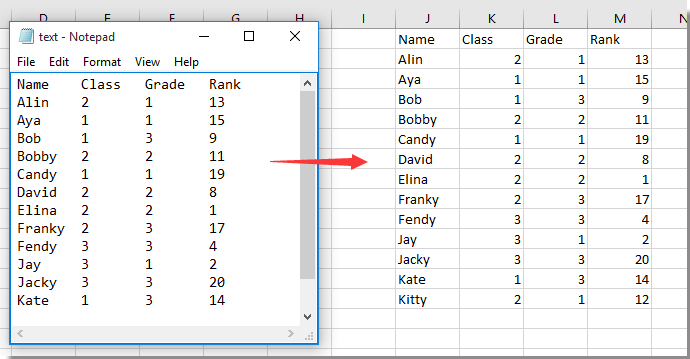### 最佳辦公生產力工具

 熱門特色: 尋找、突出顯示或識別重複項   |  刪除空白行   |  合併列或儲存格而不遺失數據   |   沒有公式的回合 ... 超級查詢: 多條件VLookup  |   多值VLookup  |   跨多個工作表的 VLookup   |   模糊查詢 .... 高級下拉列表: 快速建立下拉列表   |  依賴下拉列表   |  多選下拉列表 .... 欄目經理: 新增特定數量的列  |  移動列  |  切換隱藏列的可見性狀態  |  比較範圍和列 ... 特色功能: 網格焦點   |  設計圖   |   大方程式酒吧   |  工作簿和工作表管理器   |  資源庫 （自動文字）   |  日期選擇器   |  合併工作表   |  加密/解密單元格   |  按清單發送電子郵件   |  超級濾鏡   |   特殊過濾器 （過濾粗體/斜體/刪除線...）... 前 15 個工具集:  12 文本 工具 (添加文本, 刪除字符，...）   |   50+ 圖表 類型 (甘特圖，...）   |   40+ 實用 公式 (根據生日計算年齡，...）   |   19 插入 工具 (插入二維碼, 從路徑插入圖片，...）   |   12 轉化 工具 (數字到單詞, 貨幣兌換，...）   |   7 合併與拆分 工具 (高級合併行, 分裂細胞，...）   |   ... 和更多#### Office Tab 為 Office 帶來選項卡式界面，讓您的工作更輕鬆

• 在Word，Excel，PowerPoint中啟用選項卡式編輯和閱讀，發布者，Access，Visio和Project。
• 在同一窗口的新選項卡中而不是在新窗口中打開並創建多個文檔。
• 將您的工作效率提高 50%，每天為您減少數百次鼠標點擊！Rated 4 out of 5 · 1 ratings
This comment was minimized by the moderator on the site
the below code can split data into columns based on space or tab while importing text file to sheets. But I don't want a separate tab for each txt file i would like them all under once sheet. The information is the same format for each file. . What can be modified to allow this to be all one one sheet instead of each file imported being a new tab any and all help would be appreciated

Sub ImportTextToExcel()
'UpdatebyExtendoffice20180911
Dim xWb As Workbook
Dim xToBook As Workbook
Dim xStrPath As String
Dim xFileDialog As FileDialog
Dim xFile As String
Dim xFiles As New Collection
Dim I As Long
Dim xIntRow As Long
Dim xFNum, xFArr As Long
Dim xStrValue As String
Dim xRg As Range
Dim xArr
Set xFileDialog = Application.FileDialog(msoFileDialogFolderPicker)
xFileDialog.AllowMultiSelect = False
xFileDialog.Title = "Select a folder [Kutools for Excel]"
If xFileDialog.Show = -1 Then
xStrPath = xFileDialog.SelectedItems(1)
End If
If xStrPath = "" Then Exit Sub
If Right(xStrPath, 1) <> "\" Then xStrPath = xStrPath & "\"
xFile = Dir(xStrPath & "*.txt")
If xFile = "" Then
MsgBox "No files found", vbInformation, "Kutools for Excel"
Exit Sub
End If
Do While xFile <> ""
xFile = Dir()
Loop
Set xToBook = ThisWorkbook
On Error Resume Next
Application.ScreenUpdating = False
If xFiles.Count > 0 Then

For I = 1 To xFiles.Count
Set xWb = Workbooks.Open(xStrPath & xFiles.Item(I))
xWb.Worksheets(1).Copy after:=xToBook.Sheets(xToBook.Sheets.Count)

ActiveSheet.Name = xWb.Name

xWb.Close False
xIntRow = ActiveCell.CurrentRegion.Rows.Count
For xFNum = 1 To xIntRow
Set xRg = ActiveSheet.Range("A" & xFNum)
xArr = Split(xRg.Text, " ")
If UBound(xArr) > 0 Then
For xFArr = 0 To UBound(xArr)
If xArr(xFArr) <> "" Then
xRg.Value = xArr(xFArr)
Set xRg = xRg.Offset(ColumnOffset:=1)
End If
Next
End If
Next
Next
End If
Application.ScreenUpdating = True
End Sub
This comment was minimized by the moderator on the site
Hi, Daniel, try below code, it import all text files in one sheet named Txt.
Notice that: if the text name is the same with the exisited sheet name, the text file may be not imported.
``````Sub ImportTextToExcel2()

'UpdatebyExtendoffice20230106

Dim xWb As Workbook

Dim xToBook As Workbook

Dim xStrPath As String

Dim xFileDialog As FileDialog

Dim xFile As String

Dim xFiles As New Collection

Dim I As Long

Dim xIntRow As Long

Dim xFNum, xFArr As Long

Dim xStrValue As String

Dim xRg As Range

Dim xArr

Dim xRowL, xRowH As Integer

Dim xTxtWS, xWSD As Worksheet

Dim xTxtWS_Rg As Range

Set xFileDialog = Application.FileDialog(msoFileDialogFolderPicker)

xFileDialog.AllowMultiSelect = False

xFileDialog.Title = "Select a folder [Kutools for Excel]"

If xFileDialog.Show = -1 Then

xStrPath = xFileDialog.SelectedItems(1)

End If

If xStrPath = "" Then Exit Sub

If Right(xStrPath, 1) <> "\" Then xStrPath = xStrPath & "\"

xFile = Dir(xStrPath & "*.txt")

If xFile = "" Then

MsgBox "No files found", vbInformation, "Kutools for Excel"

Exit Sub

End If

Do While xFile <> ""

xFile = Dir()

Loop

Set xToBook = ThisWorkbook

On Error Resume Next

Set xTxtWS = xToBook.Worksheets("Txt")

If IsNull(xTxtWS) Or IsEmpty(xTxtWS) Then

xTxtWS.Name = "Txt"

End If

Application.ScreenUpdating = False

xTxtWS.Activate

If xFiles.Count > 0 Then

xRowL = 1

For I = 1 To xFiles.Count

Set xWb = Workbooks.Open(xStrPath & xFiles.Item(I))

xWb.Worksheets(1).Copy after:=xToBook.Sheets(xToBook.Sheets.Count)

Set xWSD = xToBook.Sheets(xToBook.Sheets.Count)

xTxtWS.Activate

xWb.Close False

xIntRow = xWSD.UsedRange.CurrentRegion.Rows.Count

For xFNum = 1 To xIntRow

Set xRg = xWSD.Range("A" & xFNum)

xArr = Split(xRg.Text, " ")

Set xTxtWS_Rg = xTxtWS.Cells.Range("A" & xRowL)

'        If UBound(xArr) > 0 Then

For xFArr = 0 To UBound(xArr)

If xArr(xFArr) <> "" Then

xTxtWS_Rg.Value = xArr(xFArr)

Set xTxtWS_Rg = xTxtWS_Rg.Offset(ColumnOffset:=1)

End If

Next

'        End If

xRowL = xRowL + 1

Next

xWSD.Delete

Next

End If

Application.ScreenUpdating = True

End Sub

``````
This comment was minimized by the moderator on the site
This works fine. But when it imports it renames sheets with name.txt how to make it keep only name without adding .txt extension to the sheet?
Rated 3.5 out of 5
This comment was minimized by the moderator on the site
replace line:
ActiveSheet.Name = xWb.Name
with:
ActiveSheet.Name = Left(xWb.Name,Len(xWb.Name)-4)
would remove last 4 letters from sheet name. Effectively giving me what i needed. name without .txt
Cheers
Rated 4 out of 5
This comment was minimized by the moderator on the site
Hi, thanks for your valuable VBA code.
However, I need a code for multiple txt files into 'a single sheet in the worksheet, not an individual sheet for each txt file'.
What should I edit your code for my purpose?

Thanks,
This comment was minimized by the moderator on the site
``````Sub Test()
'UpdatebyExtendoffice 10/26/2022
Dim xWb As Workbook
Dim xToBook As Workbook
Dim xStrPath As String
Dim xFileDialog As FileDialog
Dim xFile As String
Dim xFiles As New Collection
Dim I As Long
Dim J As Long
Dim xRg As Range
Dim xSaveRg As Range
Dim xSh As Worksheet

Set xFileDialog = Application.FileDialog(msoFileDialogFolderPicker)
xFileDialog.AllowMultiSelect = False
xFileDialog.Title = "Select a folder [Kutools for Excel]"
If xFileDialog.Show = -1 Then
xStrPath = xFileDialog.SelectedItems(1)
End If
If xStrPath = "" Then Exit Sub
If Right(xStrPath, 1) <> "\" Then xStrPath = xStrPath & "\"
xFile = Dir(xStrPath & "*.txt")
If xFile = "" Then
MsgBox "No files found", vbInformation, "Kutools for Excel"
Exit Sub
End If
Do While xFile <> ""
xFile = Dir()
Loop
Set xToBook = ThisWorkbook
Set xRg = xSh.Range("A1")
J = 1
Application.ScreenUpdating = False
If xFiles.Count > 0 Then
For I = 1 To xFiles.Count
Set xWb = Workbooks.Open(xStrPath & xFiles.Item(I))
Set xSaveRg = xWb.Worksheets(1).UsedRange
J = xSaveRg.Rows.Count + 1 + J
xSaveRg.Copy Destination:=xRg
On Error Resume Next
xWb.Close False

Set xRg = xSh.Cells(J, 1)
Next
End If
Application.ScreenUpdating = True
End Sub``````
This comment was minimized by the moderator on the site
In the below code if i want to specify the folder rather than selecting the path everytime import a text file , what modification have have to do

VBA CODE:

Sub ImportCSVsWithReference()
'UpdatebyKutoolsforExcel20151214
Dim xSht As Worksheet
Dim xWb As Workbook
Dim xStrPath As String
Dim xFileDialog As FileDialog
Dim xFile As String
On Error GoTo ErrHandler
Set xFileDialog = Application.FileDialog(msoFileDialogFolderPicker)
xFileDialog.AllowMultiSelect = False
xFileDialog.Title = "Select a folder [Kutools for Excel]"
If xFileDialog.Show = -1 Then
xStrPath = xFileDialog.SelectedItems(1)
End If
If xStrPath = "" Then Exit Sub
Set xSht = ThisWorkbook.ActiveSheet
If MsgBox("Clear the existing sheet before importing?", vbYesNo, "Kutools for Excel") = vbYes Then xSht.UsedRange.Clear
Application.ScreenUpdating = False
xFile = Dir(xStrPath & "\" & "*.txt")
Do While xFile <> ""
Set xWb = Workbooks.Open(xStrPath & "\" & xFile)
ActiveSheet.UsedRange.Copy xSht.Range("A" & Rows.Count).End(xlUp).Offset(1)
xWb.Close False
xFile = Dir
Loop
Application.ScreenUpdating = True
Exit Sub
ErrHandler:
MsgBox "no txt files", , "Kutools for Excel"
End Sub
This comment was minimized by the moderator on the site
``````Sub Test()
'UpdatebyExtendoffice6/7/2016
Dim xWb As Workbook
Dim xToBook As Workbook
Dim xStrPath As String
Dim xFile As String
Dim xFiles As New Collection
Dim I As Long
xStrPath = "C:\Users\AddinsVM001\Desktop\test" 'Here is the parth you can modify
If Right(xStrPath, 1) <> "\" Then xStrPath = xStrPath & "\"
xFile = Dir(xStrPath & "*.txt")
If xFile = "" Then
MsgBox "No files found", vbInformation, "Kutools for Excel"
Exit Sub
End If
Do While xFile <> ""
xFile = Dir()
Loop
Set xToBook = ThisWorkbook
If xFiles.Count > 0 Then
For I = 1 To xFiles.Count
Set xWb = Workbooks.Open(xStrPath & xFiles.Item(I))
xWb.Worksheets(1).Copy after:=xToBook.Sheets(xToBook.Sheets.Count)
On Error Resume Next
ActiveSheet.Name = xWb.Name
On Error GoTo 0
xWb.Close False
Next
End If
End Sub``````

"C:\Users\AddinsVM001\Desktop\test" is the folder path you may import text file from, please change it as you need.
This comment was minimized by the moderator on the site
The code works but imports each text file to a new tab in the workbook. Any idea where in the code this could be changed to import the new text file on the same worksheet below the data from the last text file?
This comment was minimized by the moderator on the site
i need you help i dont have any idea vba excel i want to import multiple text file like 13000. the text file name same as the cell for example (c1=112 so the text file name is also 112) mean the text file 112 is import the c112.
This comment was minimized by the moderator on the site
0

i need you help i dont have any idea vba excel i want to import multiple text file like 13000. the text file name same as the cell for example (c1=112 so the text file name is also 112) mean the text file 112 is import the c112.
This comment was minimized by the moderator on the site
Hi, my code runs but only imports the first file. It says there was a method error for copy. The debugger highlights the following line of code. Any ideas?

xWb.Worksheets(1).Copy after:=xToBook.Sheets(xToBook.Sheets.Count)
This comment was minimized by the moderator on the site
Hey Martinho,
I had the same Problem and solved it by changing this line:
Set xToBook = ThisWorkbook
to
Set xToBook = ActiveWorkbook
Maybe this helps.
This comment was minimized by the moderator on the site
thanks a lotdid the job on office 2007 excel
This comment was minimized by the moderator on the site
is there any chance for taking sheet names only certain part from txt file names?

as per above code the entire sheet name has been taking.
There are no comments posted here yet